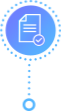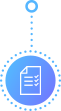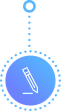The WACC was calculated to be 6.98%. Compared with a sensitivity analysis, the shares are currently undervalued at the price of \$42.09. This means that Nike shares would be a good investment for value investors like Kimi Ford and she is encouraged to invest in the shares at the current price.

Several assumptions were made during the computation. The rate of taxation was assumed to be the average of the effective tax rate for the last five years. The growth rate is assumed to remain at the predicted 5.50% for the dividends. Lastly, the computation used current yield on Nike's current debt considering that this would be the cost at which Nike would get additional debt funding if needed. The computations therefore help in ensuring that the WACC thus considers all of the current information and facts in the capital markets (Schill, Bruner & Eades, 2013).

One of the greatest inputs for a valuation of a company such as Nike is the WACC. WACC is the acronym for the weighted average cost of capital. It is computed by simply calculating the average of the costs of major sources of capital. The sources of capital include debt capital and equity capital for which equity includes preferred stock, ordinary stock, and the retained earnings. Mostly, a firm uses debt capital before considering using any other sources of capital due to the cost of capital associated with each source of capital (Schill, Bruner & Eades, 2013). This report provides a brief analysis of the cost of capital for Nike based on the provided case study. The intention is to be able to determine what the correct WACC was for Nike at the time of the case and also to be able to give advice to Kimi Ford on whether or not she should invest in Nike's shares considering the sensitivity analysis.

First Order Discount 15% OFF

Total price:

The consideration of WACC comes from the understanding that a company like Nike uses capital from various sources, which fall either under debt capital or under equity categories. The two main components of the capital are weighted in different proportions considering the leverage costs and threats as well as the company's preferences on the cost structure (Schill, Bruner & Eades, 2013). With regard to the cost structure, this is the proportionate mix of debt and equity financing and for instance, a company may have a structure of 40% debt and 60% equity meaning that the debt capital must not exceed a maximum of 40% of its total capital at any given point in time and secondly, the weight of equity should be no less than 60% at any given point in time. There are various advantages and disadvantages of using equity and debt as sources of capital but these are discusses in this report.

WACC is computed using the formula:

WACC = wD. kD + wE. kE

From the WACC equation presented above, the computation of the specific weights (wd, wr, ws) of various sources of capital is quite straight forward. However, the computation of the cost of each of the sources of capital may be a little more complex and at times confusing hence it is very easy to make mistakes if consideration is not taken. For the readers' consideration, the cost of capital can either be the cost at which the company acquired debt or the cost at which the company would acquire debt financing in the market at the time of consideration (Schill, Bruner & Eades, 2013). On the other hand, the cost of equity is assumed to be the expected return on investment as well as the cost which the company would incur in order to issue new shares of capital.

##### Want some help with your writing assignment?

The cost of debt capital is determined by the interest rates at the time of computation. This is because it is at the prevailing rates that the company would acquire debt financing from a bank or issue bonds as a sources of capital. If it is determined that the company's interest payments are in sync with the prevailing interest rates, then the interest can be used as the cost of debt. On the other hand, the cost of equity, both kr and ks are simply the required rate of return. It is computed by using the highly regarded capital asset pricing model which considers the premium that the company's members expect to get above the risk free rate. Additionally, the cost of equity can be determined using the discounted cash flow method and in this particular cases the cost of equity would be the dividends pay out divided by the market price of Nike shares at the time of valuation (Schill, Bruner & Eades, 2013).

From exhibit 5 of the case study, Cohen determined that debt comprised 27% of total capital while equity was determined to be 73%. The rate of taxation was determined to be 38%. Her computations then returned 2.7% and 10.5% as the cost of equity. Overall, the WACC was determined to be 8.4%.

Considering all of the assumptions made by Cohen, the computation of WACC was correct, which is to say that using the book value of debt and the book value of equity to compute the weights of each component at 27% and 73% respectively was correct. However, the model used could be improved by combining the use of DDM and CAPM considering that the equity component can be broken down to both retained earnings (kr) and new shares (ks). One can simply consider the cost of debt as the current yield on publicly traded Nike debt as this would be the cost at which the company would acquire new funding. The preferred stock cost of capital can be assumed to be minimal or within the scope of ke, considering the fact that the price of the preferred shares is also not provided. Using this information, the recomputed WACC can be presented as follows:

 WACC COMPUTATION WACC 6.98% Wd 11% Kd 7.06% Wr 28% Kr 6.72% Ws 61% Ks 7.08%Exclusive savings! Save 25% on your ORDER

Get 15% OFF your FIRST ORDER (code: masters15) + 10% OFF every order by receiving 300 words/page instead of 275 words/page

Considering Kimi Ford's sensitivity analysis and the MPS of the Nike shares at the time of analysis, the WACC herein computed indicates that the shares of Nike are undervalued. This is because the company's cost of capital is considerably lower compared to the findings of Cohen and it is also considerably lower than the cost admissible for the sensitivity analysis whose minimum, as indicated in exhibit 2, is 8%. Kimi Ford should invest in the shares of Nike in order to take advantage of the current low price on the market and the value of the company.STEP 2

Make the PaymentSTEP 4STEP 1

Place an OrderSTEP 3

Communicate with the Writer

In order to reach this conclusion several assumptions were made. Firstly, the growth rate will remain at the predicted 5.50%. The effective tax rate will be the average of the effective rate for the last five years (Schill, Bruner & Eades, 2013). Lastly, the current yield on Nike's current debt would be the cost at which Nike would get additional debt funding if needed.